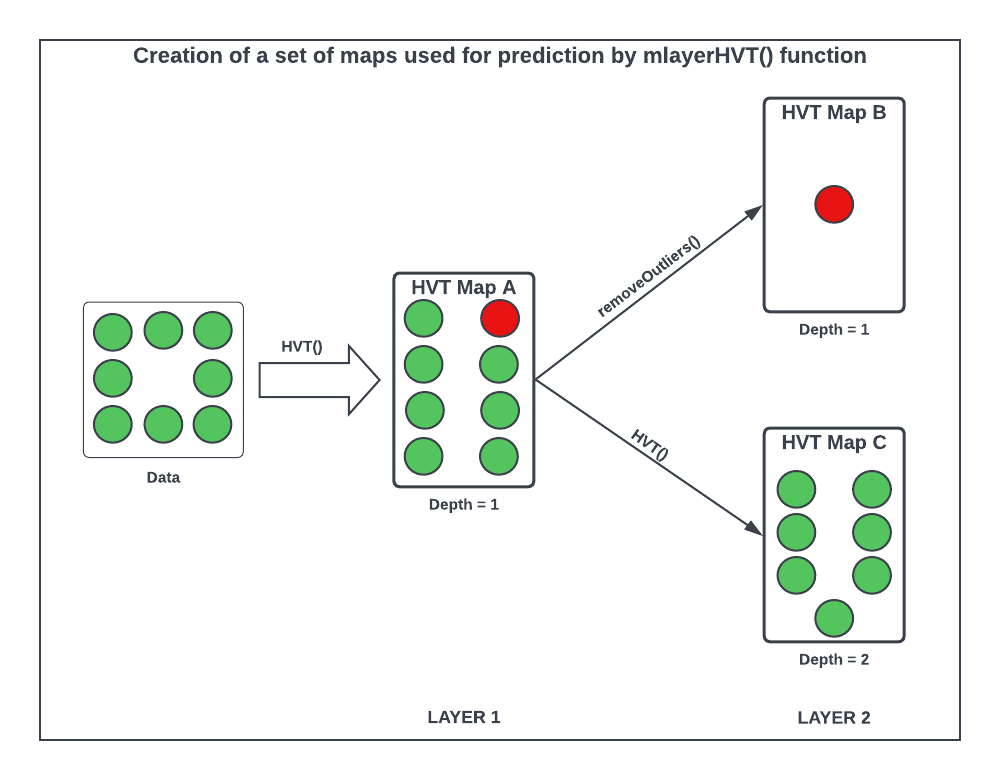# 1 Abstract

The muHVT package is a collection of R functions to facilitate building topology preserving maps for rich multivariate data. Tending towards a big data preponderance, a large number of rows. A collection of R functions for this typical workflow is organized below :

1. Data Compression: Vector quantization (VQ), HVQ (hierarchical vector quantization) using means or medians. This step compresses the rows (long data frame) using a compression objective

2. Data Projection: Dimension projection of the compressed cells to 1D,2D and 3D with the Sammons Nonlinear Algorithm. This step creates topology preserving map coordinates into the desired output dimension

3. Tessellation: Create cells required for object visualization using the Voronoi Tessellation method, package includes heatmap plots for hierarchical Voronoi tessellations (HVT). This step enables data insights, visualization, and interaction with the topology preserving map. Useful for semi-supervised tasks

4. Prediction: Scoring new data sets and recording their assignment using the map objects from the above steps, in a sequence of maps if required

This package additionally provides functions for computing Sammon’s projection and plotting the heat map of the variables on the tiles of the tessellations.

The muHVT process involves three steps:

1. Compress: Using a quantization threshold
2. Project: Using a dimension projection algorithm
3. Tessellate: Using a Voronoi Tessellation

# 2 muHVT 3.0.0 | What’s New?

06th December, 2022

This package now additionally provides functionality to predict based on a set of maps to monitor entities over time.

The creation of a predictive set of maps involves four steps -

1. Compress: Compress the datasetInstallation of muHVT 3.0.0 using a percentage compression rate and a quantization threshold using the HVT() function (Map A)
2. Remove outlier cells: Manually identify and remove the outlier cells from the dataset using the removeOutliers() function (Map B)
3. Compress the dataset without outliers: Again, compress the dataset without outlier(s) using n_cells, depth and a quantization threshold using the HVT() function (Map C)
4. Predict based on a predictive set of maps: Using the mlayerHVT() function

Let us try to understand the steps with the help of the diagram below -Figure 1: Flow diagram for predicting based on a set of maps using mlayerHVT()

Initially, the raw data is passed, and a highly compressed Map A is constructed using the HVT function. The output of this function will be hierarchically arranged vector quantized data that is used to identify the outlier cells in the dataset using the number of data points within each cell and the z-scores for each cell.

The identified outlier cell(s) is then passed to the removeOutliers function along with Map A. This function removes the identified outlier cell(s) from the dataset and stores them in Map B as shown in the diagram. The final output of this function is a list of two items - a newly constructed map (Map B), and a subset of the dataset without outlier cell(s).

The plotCells function plots the Voronoi tessellations for the compressed map (Map A) and highlights the identified outlier cell(s) in red on the plot. The function requires the identified outlier cell(s) number and the compressed map (Map A) as input in order to plot the tessellations map and highlight those outlier cells on it.

The dataset without outlier(s) gotten as an output from the removeOutliers function is then passed as an argument to the HVT function with other parameters such as n_cells, quant.error, depth, etc. to construct another map (Map C).

Finally, all the constructed maps are passed to the mlayerHVT function along with the test dataset on which the function will predict/score for finding which map and what cell each test record gets assigned to.

For detailed information on the above functions, refer the vignette.

Following are the links to the vignettes for the muHVT package:

1. muHVT Vignette: Contains descriptions of the functions used for vector quantization and construction of hierarchical voronoi tessellations for data analysis

2. muHVT Model Diagnostics Vignette: Contains descriptions of functions used to perform model diagnostics and validation for muHVT model

3. muHVT : Using mlayerHVT() for Monitoring Entities over Time Contains descriptions of the functions used for monitoring entities over time using a predictive set of HVT maps